Rectangle

Calculate perimeter of the rectangle with sides a=2.4 m and b=1.9 m.

Result

x =  8.6 m

Solution:Leave us a comment of example and its solution (i.e. if it is still somewhat unclear...):Be the first to comment!Next similar examples:

1. PlaygroundRectangular playground is fenced with 38 m long netting. Its width is 7 m. Calculate its length.
2. RectangleThe rectangle area is 182 dm2, its base is 14 dm. How long is the other side? Calculate its perimeter.
3. Ratio of perimeters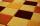Rectangle ABCD has dimensions 3 cm and 4 cm, KLMN rectangle has dimensions 4 cm and 12 cm. Calculate the ratio of the perimeter ABCD and perimeter KLMN.
4. A rectangularA rectangular garden 40 m long and 30 m wide is to be fenced with fence posts at each corner. All the other posts will be 5 meters a part. How many posts will be needed to fence in the garden.
5. Table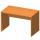Find the circumference of the table, where the long side is 1.28 meters and the short side is 86 cm.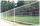Zahrada má rozměry 5m a 400cm. Kolik pletiva třeba na oplocení pozemku?
7. Foot in busIt was 102 people on the bus. 28 girls had two dogs. A 11 girls had one dog. At the next stop seceded 5 dogs (even with their owners). They got two boys together with three dogs. The bus drove one driver. How many foot were in bus?
8. Circles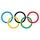For the circle c1(S1; r1=146 cm) and c2(S2; r2 = 144 cm) is distance of centers |S1S2| = 295 cm. Determine the distance between the circles.Added together and write as decimal number: LXVII + MLXIV
10. Eq1Solve equation: 4(a-3)=3(2a-5)
11. Math classificationIn 3A class are 27 students. One-third got a B in math and the rest got A. How many students received a B in math?
12. Valid numberRound the 453874528 on 2 significant numbers.
13. The resultHow many times I decrease the number 1632 to get the result 24?
14. Ten pupils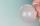10 pupils went to the store. 6 pupils bought lollipops and 9 pupils bought chewing gum. How many pupils have bought both lollipops and chewing gums (if everyone bought something)?
15. If-then equationIf 5x - 17 = -x + 7, then x =
16. Line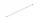How many parts of line divide 5 (different) points that lie on it?
17. CagesHonza had three cages (black, silver, gold) and three animals (guinea pig, rat and puppy). There was one animal in each cage. The golden cage stood to the left of the black cage. The silver cage stood on the right of the guinea pig cage. The rat was in the